ConicBundle

represents a polyhedral feasible ground set by affine constraints as in Linear Programming with special support for simple box constraints More...

#include <LPGroundset.hxx>

Inheritance diagram for ConicBundle::LPGroundset: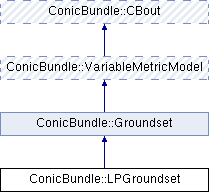## Public Member Functions

virtual void clear (CH_Matrix_Classes::Integer indim=0, CH_Matrix_Classes::Integer in_groundset_id=0)
resets all values as described in Groundset::clear()

LPGroundset (CBout *cb=0)
calls clear() with the same parameters

LPGroundset (CH_Matrix_Classes::Integer dim, const CH_Matrix_Classes::Matrix *lbyp=0, const CH_Matrix_Classes::Matrix *ubyp=0, const CH_Matrix_Classes::Sparsemat *Gp=0, const CH_Matrix_Classes::Matrix *rhslbp=0, const CH_Matrix_Classes::Matrix *rhsubp=0, const CH_Matrix_Classes::Matrix *start_val=0, const CH_Matrix_Classes::Matrix *costs=0, const CH_Matrix_Classes::Real offset=0., CH_Matrix_Classes::Integer in_groundset_id=0, CBout *cb=0)
allows to specify the groundset in the constructor, zero is allowed everywhere

CH_Matrix_Classes::Integer get_groundset_id () const
returns the current groundset_id, increased values indicate changes in the ground set

void set_groundset_id (CH_Matrix_Classes::Integer gsid)
sets the groundset_id to the desired value, increasing it is safer here because this is used to indicate changes

virtual CH_Matrix_Classes::Integer get_dim () const
returns the dimension of the ground set, i.e., the length of the variables vector y

int set_qpsolver (QPSolverParametersObject *qpparams, QPSolverObject *qpsolver=0)
Set the qp solver's parameters to qpparams (if not null); if the second argument qpsolver is also given, the old solver is first discarded and replaced by this new solver and then the parameters are set (if given). Any object passed here will be owned and deleted by *this. For correct continuaton a new qpsolver needs to have the same feasible set as the current solver but this must be ensured by the caller.

bool constrained () const
returns false if the feasible set is the entire space (unconstrained optimization), true otherwise.

const CH_Matrix_Classes::Matrixget_lby () const
number of linear constraints More...

const CH_Matrix_Classes::Matrixget_uby () const
returns the upper bounds vector on y if it exists

virtual bool is_feasible (CH_Matrix_Classes::Integer &in_groundset_id, const CH_Matrix_Classes::Matrix &y, CH_Matrix_Classes::Real relprec=1e-10)
returns the indices of variable lower bounds > ConicBundle::CB_minus_infinity More...

int ensure_feasibility (CH_Matrix_Classes::Integer &in_groundset_id, CH_Matrix_Classes::Matrix &y, bool &ychanged, BundleProxObject *Hp, CH_Matrix_Classes::Real relprec=1e-10)
makes y feasible if not so, see Groundset::ensure_feasibility()

virtual QPSolverObjectget_qp_solver (bool &solves_model_without_gs, BundleProxObject *Hp)
returns a pointer to an internal QPSolverObject that is able to solve bundle suproblems efficiently for this kind of groundset and scaling; if solves_model_without_gs == true the qp solver does not include the groundset and the groundset has to be dealt with by the Gauss Seidel approach

int set_qp_solver_parameters (QPSolverParametersObject *)
set parameters for the QP_Solver

virtual const CH_Matrix_Classes::Matrixget_starting_point () const
returns a stored starting point, note: this need not be feasible; if generated automatically, its dimension is correct.

virtual int set_starting_point (const CH_Matrix_Classes::Matrix &vec)
stores the a new starting point irrespective of whether it is feasible or not and returns 0 if it feasible, 1 if it is infeasible

virtual int candidate (CH_Matrix_Classes::Integer &gs_id, CH_Matrix_Classes::Matrix &newy, CH_Matrix_Classes::Real &cand_gs_val, CH_Matrix_Classes::Real &linval, CH_Matrix_Classes::Real &augval_lb, CH_Matrix_Classes::Real &augval_ub, CH_Matrix_Classes::Real &subgnorm2, const CH_Matrix_Classes::Matrix &center_y, CH_Matrix_Classes::Real center_value, const MinorantPointer &model_minorant, BundleProxObject *Hp, MinorantPointer *delta_groundset_minorant=0, CH_Matrix_Classes::Indexmatrix *delta_index=0, CH_Matrix_Classes::Real relprec=1e-2)
computes the next ground set minorant and candidate, see Groundset::candidate()

virtual const MinorantPointerget_gs_aggregate () const
returns the groundset aggregate computed in candidate()

const MinorantPointerget_gs_minorant () const
returns the linear minorant valid on the entire ground set (e.g. a linear cost funciton)

virtual const CH_Matrix_Classes::Indexmatrixget_yfixed () const
if not NULL (iff get_use_yfixing()==false) it returns the vector yfixed with yfixed(i)=0 if not fixed, =1 is fixed already, =2 if newly fixed

virtual CH_Matrix_Classes::Indexmatrixset_yfixed ()
if not NULL (iff get_use_yfixing()==false) returns the vector yfixed with yfixed(i)=0 if not fixed, =1 is fixed already, =2 if newly fixed

bool get_use_yfixing () const
true if the cooridinate fixing heuristic is switched on (only constrained cases)

void set_use_yfixing (bool uyf)
set to true to switch on the cooridinate fixing heuristic (only constrained cases)

int set_variable_metric_selection (VariableMetricSelection *vms=0)
delete old selector and set a new one (0 is allowed resulting in no local selector)

VariableMetricSelectionget_variable_metric_selection () const
delete old selector and set a new one (0 is allowed resulting in no local selector)

int add_variable_metric (VariableMetric &H, CH_Matrix_Classes::Integer y_id, const CH_Matrix_Classes::Matrix &y, bool descent_step, CH_Matrix_Classes::Real weightu, CH_Matrix_Classes::Real model_maxviol, const CH_Matrix_Classes::Indexmatrix *indices=0)
see VariableMetric

GroundsetModificationstart_modification ()
propagates the call to QPSolverObject::QPstart_modification() of the current qpsolver

int apply_modification (const GroundsetModification &mdf)
change the groundset description as specified by the argument

int mfile_data (std::ostream &out) const
m-file output routine for debugging or testing in Matlab (not yet working)

void set_cbout (const CBout *cb, int incr=-1)
output settingsPublic Member Functions inherited from ConicBundle::VariableMetricModel
VariableMetricModel (CBout *cb=0, int cbincr=-1)
constructor for passing on ouptut information

virtual ~VariableMetricModel ()
virtual destructor

virtual VariableMetricModelvariable_metric_transform ()
Overload this in order apply transformations in between. More...Public Member Functions inherited from ConicBundle::CBout
virtual void set_out (std::ostream *out=0, int print_level=1)
Specifies the output level (out==NULL: no output at all, out!=NULL and level=0: errors and warnings, level>0 increasingly detailed information) More...

void clear_cbout ()
reset to default settings (out=0,print_level=1)

CBout (const CBout *cb=0, int incr=-1)
calls set_cbout

CBout (std::ostream *outp, int pl=1)
initialize correspondingly

CBout (const CBout &cb, int incr=0)
copy constructor

virtual bool cb_out (int pl=-1) const
Returns true if out!=0 and (pl<print_level), pl<0 should be used for WARNINGS and ERRORS only, pl==0 for usual output.

std::ostream & get_out () const
If cb_out() returned true, this returns the output stream, but it will abort if called with out==0.

std::ostream * get_out_ptr () const
returns the pointer to the output stream

int get_print_level () const
returns the print_level

## Private Attributes

CH_Matrix_Classes::Integer dim
current dimension of the ground set

CH_Matrix_Classes::Integer groundset_id
nonnegative update counter for recognizing changes in the groundset description

CH_Matrix_Classes::Matrix starting_point
the starting point

MinorantPointer gs_aggregate
the ground set aggregate, here giving the linear coefficients of the affine cost function

bool use_yfixing
if set to true in constrained versions, y-variables that are at strongly active bounds should be fixed to this value by indicating so in yfixed

CH_Matrix_Classes::Indexmatrix yfixed
in constrained versions, when use_yfixing is true, this indicates whether a coordinate should be considered as free (0), fixed (1), or newly fixed (2)

QPSolverObjectqpsolver
general solver for all groundsets

UQPSolver uqpsolver
special solver for easy groundsets

QPSolverObjectqp_solver
the selected solver

bool solve_model_without_gs
selected varaint of solving the subproblem

BundleProxObjectHp
points to the quadratic cost matrix inside ensure_feasibility() and candidate(), otherwise ==NULL

CH_Matrix_Classes::Matrix c
linear cost term for QP-subproblems

CH_Matrix_Classes::Real gamma
constant offset for QP-subproblems

MinorantPointer gs_minorant
affine cost function for the ground set

VariableMetricSelectionvm_selection
specifieable routine for computing a metric contribution of the groundset, see Variable Metric

bool store_old_minorants
false by default, set to true if variable metric is used

MinorantBundle old_minorants
minorants collected for variable metric purpose

CH_Matrix_Classes::Integer max_minorants
maximum number of minorants

CH_Matrix_Classes::Integer minorant_nexti
used by the variable metric low rank heuristic

CH_Matrix_Classes::Matrix old_lowrank
used by the variable metric low rank heuristic

CH_Matrix_Classes::Matrix old_diagonal
used by the variable metric low rank heuristic

CH_Matrix_Classes::Symmatrix old_sym
used by the variable metric heuristic

## Detailed Description

represents a polyhedral feasible ground set by affine constraints as in Linear Programming with special support for simple box constraints

The polyhedral feasible set is described in the form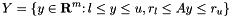where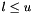are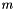-vectors,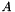is an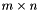matrix and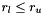are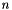-vectors.andmay be zero (empty), lower and upper bounds may take values ConicBundle::CB_minus_infinity and ConicBundle::CB_plus_infinity to implement appropriate inequalties.

For general polyhedral ground sets the two routines ensure_feasibility() and candidate() need to solve a general convex QP; this is currently done by interior point methods.

If there are only box constraints and a diagonal scaling matrix for the proximal term, then all computations are done directly witout the need to solve a full convex QP. In this case the routine also offers a scaling heuristic and a heuristic for fixing y variables at their bounds.

## ◆ get_lby()

 const CH_Matrix_Classes::Matrix* ConicBundle::LPGroundset::get_lby ( ) const

number of linear constraints

returns the lower bounds vector on y if it exists

Referenced by constrained().

## ◆ is_feasible()

 virtual bool ConicBundle::LPGroundset::is_feasible ( CH_Matrix_Classes::Integer & in_groundset_id, const CH_Matrix_Classes::Matrix & y, CH_Matrix_Classes::Real relprec = 1e-10 )
virtual

returns the indices of variable lower bounds > ConicBundle::CB_minus_infinity

returns the indices of variable lower bounds < ConicBundle::CB_plus_infinity returns the constraint matrix of the feasible set returns the constraint lower bounds returns the constraint upper bounds returns the indices with constraint lower bound slacks returns the indices with constraint upper bound slacks returns true if still feasible, see Groundset::is_feasible()

Implements ConicBundle::Groundset.

Referenced by constrained(), and set_starting_point().

The documentation for this class was generated from the following file: